Next: Exercises Up: Spin Angular Momentum Previous: Pauli Representation

# Spin Precession

According to classical physics, a small current loop possesses a magnetic moment of magnitude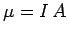, where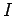is the current circulating around the loop, andthe area of the loop. The direction of the magnetic moment is conventionally taken to be normal to the plane of the loop, in the sense given by a standard right-hand circulation rule. Consider a small current loop consisting of an electron in uniform circular motion. It is easily demonstrated that the electron's orbital angular momentum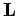is related to the magnetic momentof the loop via(758)

whereis the magnitude of the electron charge, andthe electron mass.

The above expression suggests that there may be a similar relationship between magnetic moment and spin angular momentum. We can write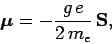(759)

where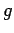is called the gyromagnetic ratio. Classically, we would expect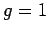. In fact,(760)

where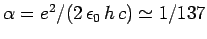is the so-called fine-structure constant. The fact that the gyromagnetic ratio is (almost) twice that expected from classical physics is only explicable using relativistic quantum mechanics. Furthermore, the small corrections to the relativistic result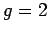come from quantum field theory.

The energy of a classical magnetic momentin a uniform magnetic fieldis(761)

Assuming that the above expression also holds good in quantum mechanics, the Hamiltonian of an electron in a-directed magnetic field of magnitudetakes the form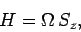(762)

where(763)

Here, for the sake of simplicity, we are neglecting the electron's translational degrees of freedom.

Schrödinger's equation can be written [see Eq. (199)](764)

where the spin state of the electron is characterized by the spinor. Adopting the Pauli representation, we obtain(765)

where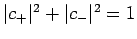. Here,is the probability of observing the spin-up state, and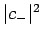the probability of observing the spin-down state. It follows from Eqs. (748), (755), (762), (764), and (765) that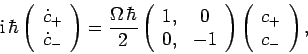(766)

where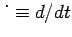. Hence,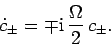(767)

Let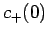(768)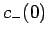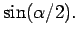(769)

The significance of the angle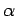will become apparent presently. Solving Eq. (767), subject to the initial conditions (768) and (769), we obtain(770)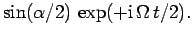(771)

We can most easily visualize the effect of the time dependence in the above expressions for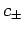by calculating the expectation values of the three Cartesian components of the electron's spin angular momentum. By analogy with Eq. (192), the expectation value of a general spin operatoris simply(772)

Hence, the expectation value ofis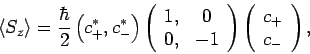(773)

which reduces to(774)

with the help of Eqs. (770) and (771). Likewise, the expectation value ofis(775)

which reduces to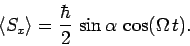(776)

Finally, the expectation value of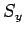is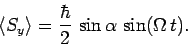(777)

According to Eqs. (774), (776), and (777), the expectation value of the spin angular momentum vector subtends a constant anglewith the-axis, and precesses about this axis at the frequency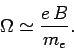(778)

This behaviour is actually equivalent to that predicted by classical physics. Note, however, that a measurement of,, orwill always yield either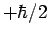or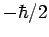. It is the relative probabilities of obtaining these two results which varies as the expectation value of a given component of the spin varies.

SubsectionsNext: Exercises Up: Spin Angular Momentum Previous: Pauli Representation
Richard Fitzpatrick 2010-07-20